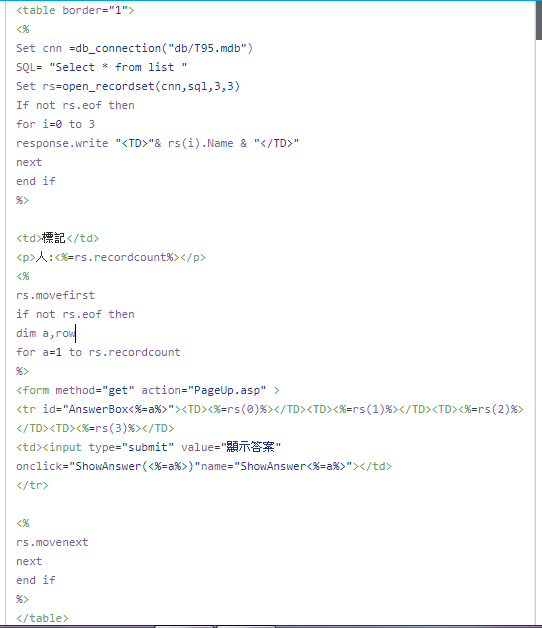#0

## asp 讀取某寫入某列欄位

1 A EE 1
2 B SCI 2
3 C SCS 3<%

c=request.servervariables("Query_String")

k=mid(c,11,3)

if right(k,1)="="then

q=replace(k,"=","")

s=Cstr(q)

else

q=replace(k,"=%","")

s=Cstr(q)

end if

Set cnn =db_connection("db/T95.mdb")

SQL= "Select * from list where 識別碼="&s&""

Set rs=open_recordset(cnn,sql,3,3)

SQL= "UPDATE list set return = 'return' where 識別碼="&s&""

response.write s

%>

SQL= "UPDATE list SET return=您要寫入的值 WHERE 識別碼=Query_String"
mayyola iT邦新手 1 級 ‧ 2017-11-08 23:04:51 檢舉

mayyola iT邦新手 1 級 ‧ 2017-11-09 08:43:50 檢舉

### 2 個回答

0

``````Set cnn =db_connection("db/T95.mdb")

SQL= "UPDATE list set return = 'return' where 識別碼="&s&""

cnn.Execute(SQL)
``````

``````response.Redirect("程式1.asp")
``````
mayyola iT邦新手 1 級 ‧ 2017-11-09 17:37:52 檢舉

f大已經可以了!謝謝0

1.
if right(k,1)="="then
q=replace(k,"=","")
else
q=replace(k,"=%","")
end if

2.這段程式碼只寫到連結db，卻沒有寫到table內值的程式碼？
update 時以 where 條件指定是哪一列要被更新，

mayyola iT邦新手 1 級 ‧ 2017-11-08 10:50:55 檢舉